FAIRFIELD UNIVERSITY

BEI School of Engineering

# Electrical Engineering Department

SYLLABUS - EE212- Computer Aided Circuit Analysis

Prerequisite: Introduction to Circuits (or equivalent)                     2 Credits        30 hours

Description:  After a brief review of fundamental circuit analysis techniques, both time and frequency domain analyses of passive and active circuits are examined. Excel spreadsheets are used to aid in computation and graphical plots. MicroSim PSpice or Electronic Workbench or a similar application is used as the basic circuit analysis program.  Use MatLab MathCAD to solve circuit problems and plot results.  The course focuses on using four different computer programs to solve circuit analysis and design problems.  Comparisons are made to assist the student in selecting the appropriate tool to accomplish a given objective.

 Instructor: Jeffrey N. Denenberg Email: jdenenberg@fair1.fairfield.edu Home Page: http://pages.cthome.net/denenberg/ Phone: (203) 268-1021(days & eves.) Textbook: “Electric Circuits,” 5th Ed., Nilsson & Riedel, 1996, Addison-Wesley Publishing Company, Inc., ISBN: 0-201-84709-4 SW: MatLab,  Excel, MathCAD, PSpice (MatLab and PSpice are supplied by instructor at no cost) Pre-Requisites: A first course in Circuit Analysis Exams: Three (~4th , 9th &14th wk) - 60%. Comprehensive final - 40%

References:

1.      Introduction to Electric Circuits, Dorf & Svoboda,  1996, John Wiley & Sons. ISBN  0-471-12702-7

2.      Electrical Circuit Analysis 2nd Edition - Johnson,  Johnson & Hilburn - 1992 - Prentice Hall, ISBN 0-13-249335-7

3.      Circuit Analysis for Engineers, Mix & Schmitt  1985,     John Wiley & Sons

4.      Circuits, Devices and Systems  5th Edition - Smith & Dorf,  John Wiley & Sons Inc,  1992            ISBN  0-471-83944-2

Outcomes

##### Learning Goals

1.

Gain confidence with PCs for engineering analysis purposes.

Use

Modern Engineering Tools

1.0

2.

Begin to understand computer strengths and limitations

3.      3.

Gain familiarity with four fundamental software applications MicroSim PSpice or Electronic Workbench, EXCEL, MatLab and MathCAD

4.

Strengthen understanding of basic circuit analysis techniques

Technical Content

0.5

5.

Apply Calculus to circuit analysis problems

Math, Science & Applications

0.5

Prepared By: Dr. J. N. Denenberg                                                  June 10, 1999

 Class Topic Text Reference 1. Course Goals, Review Kirchhoff’s Laws, Review Mesh & Nodal Analysis, Introduction to MatLab Chap 1, 2, 3 1.1. Thevenin & Norton Theorems Chap. 4 2. Phasor Analysis.  Complex numbers.  Use of  EXCEL in solving EE Problems - EXCEL Usage Lab Chap 9 3. Frequency Domain Analysis - EXCEL Usage Lab 4. Mid-term Exam.  Operational Amplifiers Chap 5 5. MicroSim OR ELECTRONIC WORKBENCH Introduction, Create & Edit Circuits. Examples RC Transient & Frequency Analysis 5.1. MicroSim OR ELECTRONIC WORKBENCH (cont'd)- RLC (Resonance),  Notch Filter, Operational Amplifier - Lab 6. Distribute Problem set and begin analysis & design. - Lab 6.1. Programming in MatLab 7. Intro. to MathCAD - Lab MathCAD Tutorial 8. Examples of MathCAD applications in EE - Lab MathCAD & Notes 9. Final Exam (Optional) 10. If time permits:  Transient Analysis  Source Free Chap 7, 8

The problem set will consist of approximately ten problems to be solved using EXCEL, PSPICE, and MathCAD or MatLab.  At the instructor’s option, the problem set may replace the Final Exam.  Design problems will be included in the problem set.

Midterm Exam                       20%

Design Assignments            10%

Class Performance  10%

Total                            100%

CLASS EXPECTATIONS

I. TEACHER

Distribute syllabus.

Review the material described in the syllabus.

Explain material.

Identify alternate reading assignments or books that clarify the material.

Relate material to "real world" situations when possible.

Meet at a mutually convenient time to discuss problems.
(I will be in the office on Thursday Evenings for the summer)

Be receptive to new ideas.

Make up missed classes.

Assign appropriate home problems.

Homework policy

Reviewed in class

Collected or not collected

Quizzes

II. STUDENT

Stay current.

Study the material described in the syllabus.

Complete the assigned homework.

Obtain class notes and homework if a class is missed.

Use the library to obtain supplemental material that explains an unclear topic.

Prepare for exams.

ANTICIPATED POSTPONED CLASS DATES

None

MAKEUP CLASS DATES

To be announced

1999 Class Meetings: May 24; June 7, 14, 21, 28; July 5, 12, 19, 26; Final Exam: August 2.

Kirchoff’s Laws

1.                  Voltage

1.1.           The Voltage around any closed path is zero.

or

1.2.           The sum of the voltage rises equals the sum of the voltage falls around a closed path.

2.                  Current

2.1.           The sum of the current at any node equals zero.

or

2.2.           The sum of the currents into a node equals the sum of the current out of the node.

---------------------------------------------------------------------------------------------------------

Thevenin Equivalent Circuit

All circuits or portions of circuits can be replaced by a Thevenin voltage source in series with a Thevenin impedance.

Do the following to perform this operation:

1.      Calculate the voltage at the points of interest to find the Thevenin equivalent voltage source.

2.      Thevenin equivalent impedance -- Set all independent sources to zero by doing the following: Short circuit all independent voltage sources and open circuit all independent current sources. Then calculate the impedance at the points of  interest  to find the Thevenin equivalent impedance.

Norton Equivalent Circuit

All circuits or portions of circuits can be replaced by a Norton current source in parallel with a Norton admittance/impedance.

Do the following to perform this operation:

1.      Short-circuit the points of interest.  Calculate the current in the short circuit to find the Norton equivalent current source value.

2.      Norton equivalent admittance/impedance -- Set all independent sources to zero by doing the following: Short-circuit all independent voltage sources and open circuit all independent current sources. Then calculate the admittance or impedance at the points of interest to find the Norton equivalent admittance or impedance.

PASSIVE  DEVICES

VIEW                          IMPEDANCE                        TIME               FREQUENCY            LAPLACE                  SERIES                      PARALLEL

DOMAIN         DOMAIN                     DOMAIN                      SUM                           SUM

--------------------------------------------------------------------------------------------------------------------------------------------------------------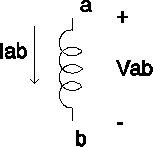INDUCTOR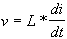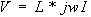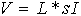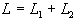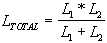Z = jwL

--------------------------------------------------------------------------------------------------------------------------------------------------------------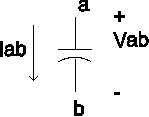CAPACITOR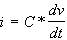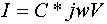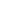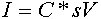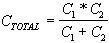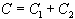Z = 1/jwC

--------------------------------------------------------------------------------------------------------------------------------------------------------------

H. Hoffman, 1/98

PASSIVE  DEVICES

VIEW                          IMPEDANCE                        TIME               FREQUENCY            LAPLACE                    SERIES                      PARALLEL

DOMAIN         DOMAIN                     DOMAIN                     SUM                           SUM

--------------------------------------------------------------------------------------------------------------------------------------------------------------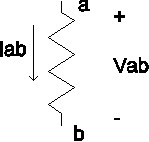RESISTOR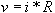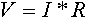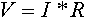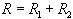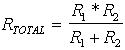Z = R

--------------------------------------------------------------------------------------------------------------------------------------------------------------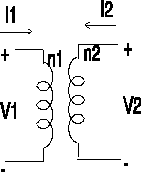TRANSFORMER      POWER IN = POWER OUT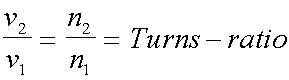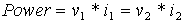H. Hoffman, 1/98

FAIRFIELD UNIVERSITY

BEI School of Engineering

Electrical Engineering Department

EE 212 Computer Aided Circuit Analysis                                             Typical Problem Set

Take Home

========================================================================

All problems must be submitted before the end of the term.  If the entire problem set is not submitted, the student will receive an incomplete grade.

========================================================================

1.  a)  Find the transfer function (V2/V1)  for the following bandpass filter .  Use phasor notation.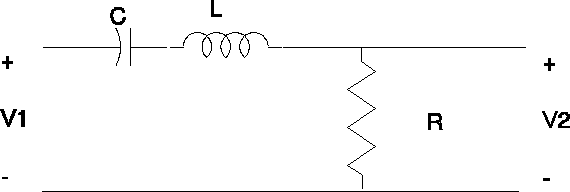b)  Determine the magnitude and phase equations for the circuit.

c)  Sketch the magnitude and phase for component values:  R= 10 Ohms,  L= 5 milliHenries,  and C= 10 mF.

d) Confirm your sketch by using EXCEL to plot the magnitude and phase

e)  Again confirm your sketch by using MicroSim or Electronic Workbench to plot the magnitude and phase.

f) Apply a periodic square wave with the following  characteristics to the input ( v1(t) ):

Rise & Fall time                     10 nanosec

zero level                                0 Volts

High level                               15 Volts

Period                                                20 milli sec.

Use MicroSim or Electronic Workbench to find v2(t)  .

g)  For the same periodic square wave described in part (f) , let R vary from 100 W to 1000 W

in steps of 100 W.

Again use MicroSim or Electronic Workbench to plot  v2(t)  for the different resistor values.

2.   Assume switch S1 is closed for t<0.  Find v(t) for t>0.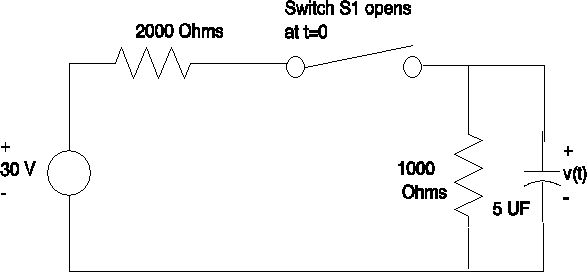3. a)  Find the transfer function (V2/V1)  for the following highpass filter.  Assume an ideal Operational amplifier.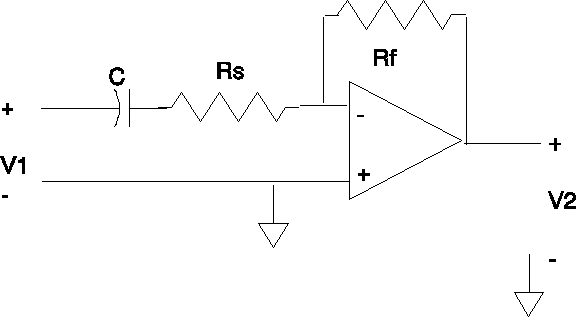b)  Determine the magnitude and phase equations for the above circuit.

c)  Sketch the magnitude and phase with the following assumptions:  Rf/Rs  = 10, and 1/RsC = 1.

d) Select values of Rf, Rs, and C that will result in a break point frequency of 1 Khz.  Verify your result by plotting the result using MicroSim or Electronic Workbench.    Identify how you would determine the break point frequency.

4.   Plot the magnitude, magnitude in dB. and phase versus of the parallel T filter circuit in Figure 1.  Use the MicroSim or Electronic Workbench program to compute the requested characteristics.  Note that you will need a jumper connection, which may be found as a connector in the component listing.  Carefully consider the frequency range over which to plot the response.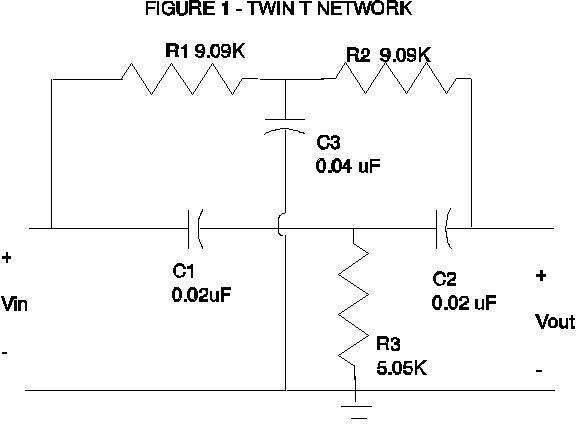5.  Given the following homogenous differential equation that describes the natural response for a circuit,

d2v              dv

---       +    3 ---         +    2  v    =   0

dt2                      dt

How many storage elements are there in the circuit?

6.  Design Problem #1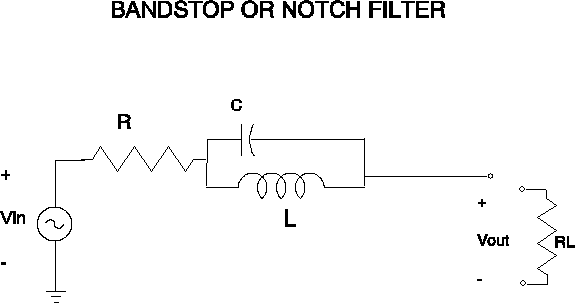Consider a bandstop filter of the form shown above.  Design a filter to reject a 60 Hz sinusoid while passing other frequencies.  The bandwidth of the rejection desired is approximately 30 Hz., the load resistor (RL ) is 600 W , and resistor (R) is 600 W.

a)     Show that the transfer function Vout/Vin is

Vout/Vin = RL /  (R+Z+ RL)  where Z is the impedance of the parallel capacitor and inductor.

b)     The resonant frequency is 60 Hz.  Using phasor notation show that for w<<  w0  and for           w0   >> w  the transfer unction for Vout/Vin  is approximately  RL /  (R+ RL)  .

c)      Organize the transfer function into the following format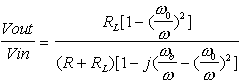where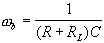wb  approximates the circuit bandwidth.

d)     The resonant frequency (w0 )  is 60 Hz.   Show that for w<<  w0  and for    w0   >> w  the transfer function for Vout/Vin  is approximately RL /  (R+ RL).

e)     Calculate the capacitor and inductor values required to implement the 60 Hz bandstop filter.

Using MicroSim or Electronic Workbench,  verify the calculation by plotting the Magnitude response.  Also plot the phase response.

7. Design Problem #2

In the previous problem, the notch filter used only passive elements.  The passive circuit may require the use of large, heavy, and expensive inductors.  The active filter below uses resistors, capacitors and op amps, but no inductors.  Select values of resistors and capacitors that will approximate a 60 Hz notch filter and a 30 Hz bandwidth for the operational amplifier bandstop filter.  Use MicroSim or Electronic Workbench to assist you in this exploration.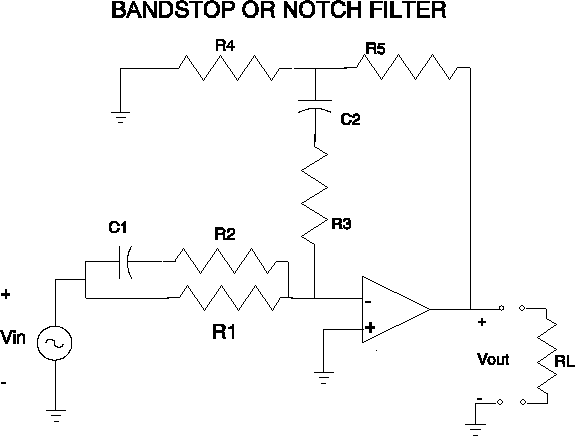8. a) Using MathCAD, calculate and plot the magnitude and phase functions for  the RLC circuit below .  Let R=10 W ,  L  = 5 milliHenries, and C = 5  mF.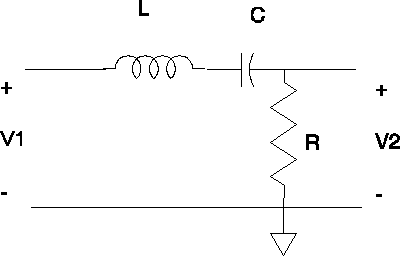Transfer Function = V2/V1

b)  Suppose we define a Quality factor, Q ,where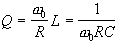.  Express the transfer function for the series resonant circuit as a function of Q,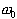, and  w .   Show that the bandwidth is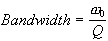.  Recall that the resonant frequency is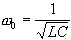.

c) Suppose we wish to design a filter with a center frequency of 1 Khz. and a bandwidth of 100 Hz.  Further assume that the load resistor, R, is  50 W.  Select the values of L and C that will provide the desired response.  Plot the magnitude of the transfer function.  You are at liberty to use any computer tools that you wish.